# Characteristics of Sensors

Sensors are characterized depending on the value of some of the parameters. Important characteristics of sensors and transducers are listed below:

• Input characteristics
• Transfer characteristics
• Output characteristics

## Input Characteristics of Sensors

1. Range: It is the minimum and maximum value of physical variable that the sensor can sense or measure. For example, a Resistance Temperature Detector (RTD) for the measurement of temperature has a range of -200 to 800oC.
2. Span: It is the difference between the maximum and minimum values of input. In above example, the span of RTD is 800 – (-200) = 1000oC.
3. Accuracy: The error in measurement is specified in terms of accuracy. It is defined as the difference between measured value and true value. It is defined in terms of % of full scale or % of reading.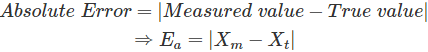Xt is calculated by taking mean of infinite number of measurements.
4.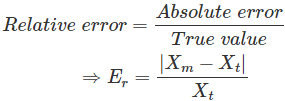5. Precision: It is defined as the closeness among a set of values. It is different from accuracy. Let Xt be the true value of the variable X and a random experiment measures X1, X2, …. Xi as the value of X. We will say our measurements X1, X2,… Xi are precise when they are very near to each other but not necessarily close to true value Xt. However, if we say X1, X2,… Xi are accurate, it means that they are close to true value Xt and hence they are also close to each other. Hence accurate measurements are always precise.
6.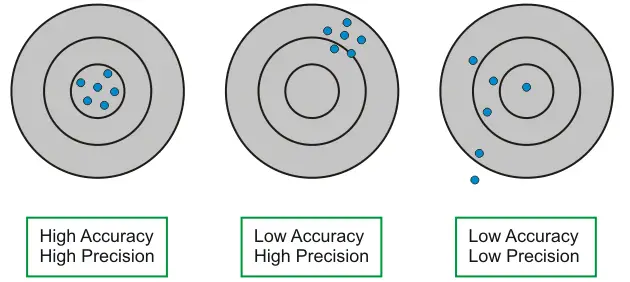7. Sensitivity: It is the ratio of change in output to change in input. If Y be the output quantity in response to input X, then sensitivity S can be expressed as
8.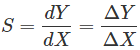9. Linearity: Linearity is the maximum deviation between the measured values of a sensor from ideal curve.
10.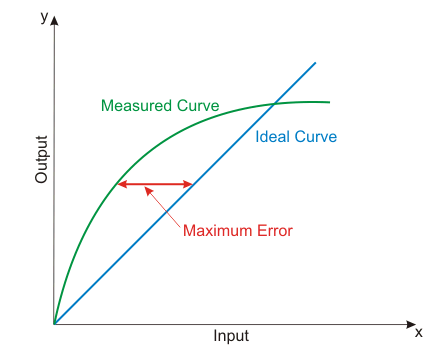11. Hysteresis: It is the difference in output when input is varied in two ways- increasing and decreasing.
12.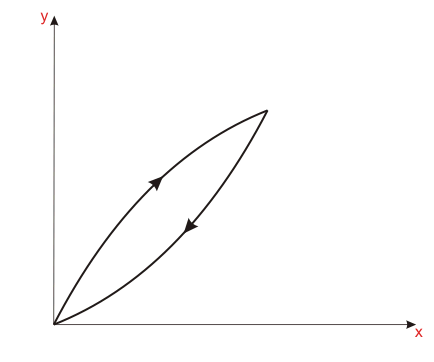13. Resolution: It is the minimum change in input that can be sensed by the sensor.
14. Reproducibility: It is defined as the ability of sensor to produce the same output when same input is applied.
15. Repeatability: It is defined as the ability of sensor to produce the same output every time when the same input is applied and all the physical and measurement conditions kept the same including the operator, instrument, ambient conditions etc.
16. Response Time: It is generally expressed as the time at which the output reaches a certain percentage (for instance, 95%) of its final value, in response to a step change of the input.

Want To Learn Faster? 🎓
Get electrical articles delivered to your inbox every week.
No credit card required—it’s 100% free.